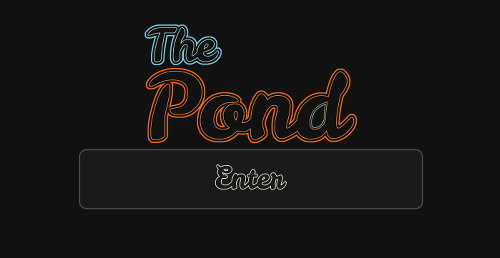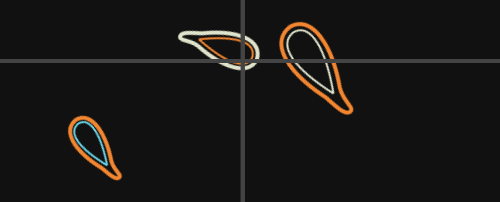# The Pond – building a multi-platform HTML5 game## Introducing The Pond

The Pond is a multi-platform HTML5 game (source code) that explores minimalistic design and resolution independent gameplay. The Pond isn’t about reaching a high score, or about buying weapon upgrades. It’s about relaxing and exploring a beautiful world.

It is available on all these platforms/in all these stores:

In making The Pond I came across many performance obstacles which I will explore in detail (especially when optimizing the codebase for mobile).

## Tools

Before I begin, I would like to mention the two tools that made coding The Pond both efficient and highly enjoyable: Light Table and CocoonJS.

Light Table is an IDE (still in alpha) which provides an integrated development environment for real-time javascript code injection. This means that javascript edited within the editor can be previewed without reloading the page. If we look at the shape of the fish in the game we notice that it is comprised of Bézier curves. Instead of trying to find an editor for creating Bézier curves, I simply estimated a basic shape and modified the variables in real-time until I was satisfied with it’s look and feel.

CocoonJS on the otherhand provides a canvas optimized compatibility layer for improved performance on mobile devices. Not only does it optimize, it also provides an interface for exporting our application to many devices (Android, iOS, Amazon (android), Pokki, and Chrome Web Store).

## Physics

The Pond may seem simple on the outside, but on the inside it’s full of performance optimizations and responsive features. As we resize the game, it updates and re-optimizes itself to render less objects and spawn less fish, and if that’s not enough the framerate degrades smoothly to keep physics in check. This is thanks to the use of a fixed interval physics time step. Gameprogrammingpatterns.com provides a good explanation for how to do this and why it matters, but honestly the code makes the most sense:

``` var MS_PER_UPDATE = 18; // Time between physics calculations var lag = 0.0; // accumulate lag over frames var previousTime = 0.0; // used for calculating the time delta   // main game loop function draw(time) { requestAnimFrame(draw); // immidiately queue another frame lag += time - previousTime; // add time delta previousTime = time;   var MAX_CYCLES = 18; // prevent infinite looping/hanging on slow machines   // physics calculations while(lag >= MS_PER_UPDATE && MAX_CYCLES) {   // user input, movement, and animation calculations physics(); lag -= MS_PER_UPDATE; MAX_CYCLES--; }   // if we exhausted our cycles, the client must be lagging if(MAX_CYCLES === 0) {   // adaptive quality lowerQuality(); }   // if 5 frames behind after update, jump // this prevents an infinite input lag from ocurring if(lag/MS_PER_UPDATE > 75) { lag = 0.0; }   // draw to canvas paint(); } ```

What’s important to notice here is that physics is not calculated based on the time delta, instead it’s calculated at a fixed 18ms interval. This is important because it means that any client lag will not be reflected in physics calculations, and that slower machines will simply lose framerate.

### Dynamic Quality

The next optimization we notice is the `lowerQuality()` function, which adaptively decreases the render quality of the game. The way this works is simply by re-sizing the drawing canvas (it’s still full screen, it simply gets streched out), which in-turn leads to reduced spawns and collisions.

``` function resizeWindow() {   // quality is a global variable, updated by lowerQuality() \$canv.width = window.innerWidth * quality/10 \$canv.height = window.innerHeight * quality/10 ctx = \$canv.getContext('2d') ctx.lineJoin = 'round'   // resize HUD elements, and reduce spawning if(GAME.state === 'playing') { GAME.spawner.resize(\$canv.width, \$canv.height) GAME.levelBar.resize(\$canv.width, \$canv.height) GAME.levelBalls.resize(\$canv.width, \$canv.height) } else { if(ASSETS.loaded) drawMenu() } } ```

### Spawning

Now, we’ve been talking about reducing spawning to improve performance so let me explain how that happens. The spawning algorithm works by creating a virtual grid sized based on the window size. As the player travels from one grid zone to another, the adjacent zones are populated with enemies:``` Spawner.prototype.spawn = function(zone) { // spawn 1-3 fish per 500sqpx, maybe larger maybe smaller than player // 0.5 chance that it will be bigger/smaller var mult = this.width*this.height/(500*500) for(var i=0, l=(Math.floor(Math.random()*3) + 1) * mult; i < l; i++) {   // spawn coordinates random within a zone var x = zone+Math.floor(this.width*Math.random()) - this.width/2 var y = zone+Math.floor(this.height*Math.random()) - this.height/2 var size = Math.random() > 0.5 ? this.player.size + Math.floor(Math.random() * 10) : this.player.size - Math.floor(Math.random() * 10)   // spawn a new fish var fish = new Fish(true, x, y, size, Math.random()*Math.PI*2-Math.PI, Math.random()*Math.PI)   this.fishes.push(fish) } return zone } ```

The last piece of the puzzle is removing enemies as they move far enough away:

``` // if far enough away from player, remove if(distance(fish, player) > Math.max(\$canv.width, \$canv.height) * 2) { fish.dead = true } ```

### Collisions

The next performance optimization lies with the collision code. Colliding irregularly shaped objects can be extremely difficult and resource intensive. One option is to do color based collision (scan for overlapping colors), but that is much too slow. Another option might be to mathematically calculate Bézier curve collisions, however this is not only CPU intensive, it is also quite difficult to code. I finally opted for an approximation approach using circles. Basically I calculate the position of circles within each fish and detect circle collision among the fish. Boolean circle collision is extremely efficient, as it simply requires measuring the distance between objects. This ends up looking like this (debug mode):``` Fish.prototype.collide = function (fish) {   // the fish has been killed and is being removed or it is far away if (this.dying || fish.dying || distance(this, fish) > this.size * 5 + fish.size*5) { return false }   // there are 6 circles that make up the collision box of each fish var c1, c2 for (var i=-1, l = this.circles.length; ++i < l;) { c1 = this.circles[i] for (var j=-1, n = fish.circles.length; ++j < n;) { c2 = fish.circles[j]   // check if they touch if(distance(c1, c2) <= c2.r + c1.r) { return true } } }   return false } ```

We also avoid unnecessary collision calculations by only checking the fish that are visible (or near-visible):

``` if(Math.abs(fish2.x - player.x) < \$canv.width && Math.abs(fish2.y - player.y) < \$canv.height) { // check } ```

## Drawing

After getting the physics+ out of the way, it’s time to optimize drawing operations. Many games use sprite maps for animation (Senshi for example) which can be highly optimized. Unfortunately our fish are dynamically generated so we must find other ways to optimizing drawing. First lets use Chrome’s javascript profiler to identify bottlenecks:What we see here is that `stroke` is using a lot of resources. Truth be told, `fill` used to be there too. This is because both were called heavily when drawing fish. The game looked a bit like this:After removing `fill` I saw a huge performance increase, and the game looked much better. The reason the `drawImage` function is up there as well is because I take advantage of offscreen canvas rendering. Each fish is drawn on its own offscreen canvas which is then rendered onto the larger visible canvas. This is also what allowed me to easily explode the fish into particles by reading pixel data:

``` Fish.prototype.toParticles = function(target) { var particles = []   // read canvas pixel data var pixels = this.ctx.getImageData(0,0,this.canv.width, this.canv.height).data for(var i = 0; i < pixels.length; i += 36 * Math.ceil(this.size/20) * (isMobile ? 6 : 1)) { var r = pixels[i] var g = pixels[i + 1] var b = pixels[i + 2]   // black pixel - no data if(!r && !g && !b){ continue }   // Math to calculate position var x = i/4 % this.canv.width - (this.canv.width/2 + this.size) var y = Math.floor(i/4 / this.canv.width) - (this.canv.height/2) var relativePos = rot(x, y, this.dir) x=this.x + relativePos y=this.y + relativePos   var col = new Color(r, g, b) var dir = directionTowards({x: x, y: y}, this) particles.push(new Particle(x, y, col, target, Math.PI*Math.random()*2 - Math.PI, this.size/20)) } return particles } ```

## The End

In the end the performance optimizations paid off and made the game feel more polished and playable even on lower-end mobile devices.

If you enjoyed this post, I regularly blog about my development projects over at http://zolmeister.com.

The Pond awaits exploring…

View full post on Mozilla Hacks – the Web developer blog

### 2 thoughts on “The Pond – building a multi-platform HTML5 game”

1.Nathan Campos

Awesome game, I loved it.

For some reason the Firefox Marketplace says that it wasn’t available in my region (Brazil).